# CASIO

User´s Guide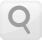## Fraction Calculations

Example 1: 23 + 15 = 1315

• 2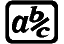3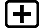15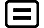•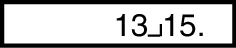Example 2: 314 + 123 = 41112

• 314123•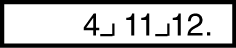Example 3: 12 + 1.6 = 2.1

• 121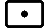6• 2.1

### Note

Values are displayed in decimal format automatically whenever the total number of digits of a fractional value (integer + numerator + denominator + separator marks) exceeds 10.

Results of calculations that mix fraction and decimal values are always decimal.

### Decimal ↔ Fraction Conversion

To switch a calculation result between fraction and decimal format:
Press.

Example 1: 2.75 = 234 (Decimal → Fraction)

• 275•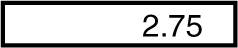••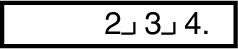• = 114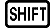(d/c)
•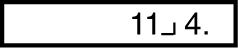Example 2: 12 ↔ 0.5 (Fraction ↔ Decimal)

• 12•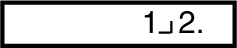••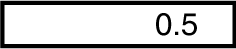••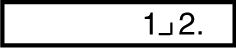### Mixed Fraction ↔ Improper Fraction Conversion

To switch a calculation result between improper fraction and mixed fraction format:
Press(d/c).

Example 1: 12353

• 123•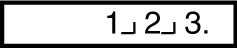•(d/c)
•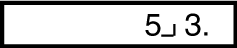•(d/c)
•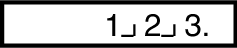### Note

You can use the display setup (Disp) screen to specify the display format when a fraction calculation result is greater than one.

To change the fraction display format, press the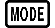key a number of times until you reach the setup screen shown below.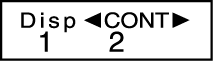Display the selection screen.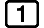(Disp)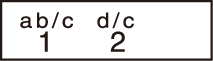Press the number key (or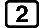) that corresponds to the setting you want to use.(ab/c): Mixed fraction(d/c): Improper fraction

An error occurs if you try to input a mixed fraction while the d/c display format is selected.